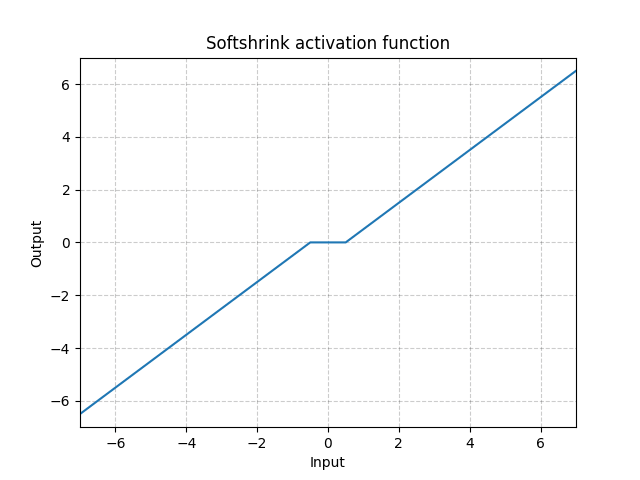/PyTorch

# Softshrink

class torch.nn.Softshrink(lambd: float = 0.5) [source]

Applies the soft shrinkage function elementwise:

$\text{SoftShrinkage}(x) = \begin{cases} x - \lambda, & \text{ if } x > \lambda \\ x + \lambda, & \text{ if } x < -\lambda \\ 0, & \text{ otherwise } \end{cases}$
Parameters

lambd – the $\lambda$ (must be no less than zero) value for the Softshrink formulation. Default: 0.5

Shape:
• Input: $(N, *)$ where * means, any number of additional dimensions
• Output: $(N, *)$ , same shape as the inputExamples:

>>> m = nn.Softshrink()
>>> input = torch.randn(2)
>>> output = m(input)


© 2019 Torch Contributors
Licensed under the 3-clause BSD License.
https://pytorch.org/docs/1.7.0/generated/torch.nn.Softshrink.html